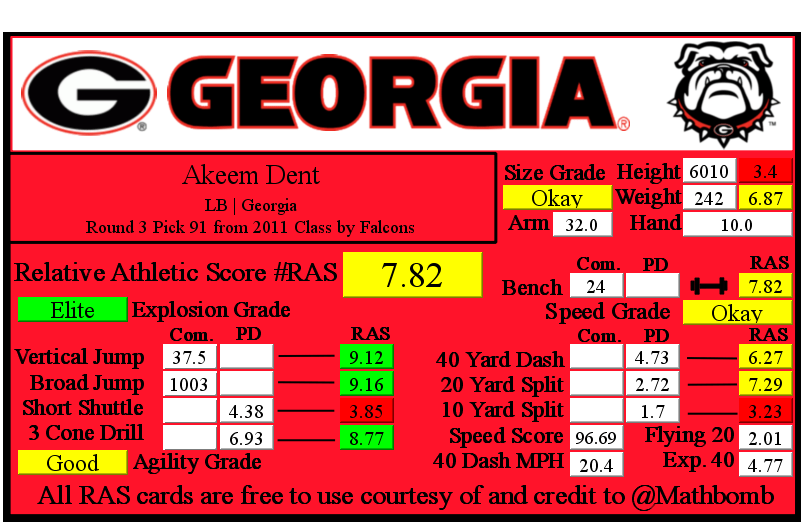# Akeem Dent RAS

### Akeem Dent RASAkeem Dent was drafted by Falcons with pick 91 in round 3 in the 2011 NFL Draft out of Georgia.

He recorded a Relative Athletic Score of 7.82, out of a possible 10.0. RAS is a composite metric on a 0 to 10 scale based on the average of all of the percentile for each of the metrics the player completed either at the Combine or pro day.

He had a recorded height of 6010 that season, recorded as XYYZ where X is feet, YY is inches, and Z is eighths of an inch. That correlates to 6 feet, 1 and 0/8 of an inch or 73.0 inches, or 185.42000000000002 centimeters. This correlates to a 3.4 score out of 10.0.

He recorded a weight of 242 in pounds, which is approximately 110 kilograms. This correlates to a 6.87 score out of 10.0.

Based on his weight, he has a projected 40 yard dash time of 4.77. This is calculated by taking 0.00554 multiplied by his weight and then adding 3.433.
This player did not have a recorded 40 yard dash for the Combine in the RAS database.

At his pro day, he recorded a 40 yard dash of 4.73 seconds. This correlates to a 6.27 score out of 10.0.

Using Bill Barnwell’s calculation, this Combine 40 time gave him a Speed Score of 96.69

The time traveled between the 20 and 40 yard lines is known as the Flying Twenty. As the distance is also known, we can calculate the player’s speed over that distance. The time he traveled the last twenty yards at his pro day was 2.01 seconds. Over 20 yards, we can calculate his speed in yards per second to 9.95. Taking into account the distance in feet (60 feet), we can calculate his speed in feet per second to 29.85. Breaking it down further, we can calculate his speed in inches per second to 358.21. Knowing the feet per second of 29.85, we can calculate the approximate miles per hour by multiplying that value by 0.681818 to give us a calculated MPH of 20.4 in the last 20 yards of his run.

This player did not have a recorded 20 yard split for the Combine in the RAS database.

At his pro day, he recorded a 20 yard split of 2.72 seconds. This correlates to a 7.29 score out of 10.0.

We can calculate the speed traveled over the second ten yards of the 40 yard dash easily, as the distance and time are both known. The time he traveled the second ten yards at his pro day was 1.02 seconds. Over 10 yards, we can calculate his speed in yards per second to 9.8. Taking into account the distance in feet (30 feet), we can calculate his speed in feet per second to 29.41. Breaking it down further, we can calculate his speed in inches per second to 352.94. Knowing the feet per second of 29.41, we can calculate the approximate miles per hour by multiplying that value by 0.681818 to give us a calculated MPH of 20.1 in the second ten yards of his run.

This player did not have a recorded 10 yard split for the Combine in the RAS database.

At his pro day, he recorded a 10 yard split of 1.7 seconds. This correlates to a 3.23 score out of 10.0.

The time he traveled the first ten yards at his pro day was 1.7 seconds. Over 10 yards, we can calculate his speed in yards per second to 6.0. Taking into account the distance in feet (30 feet), we can calculate his speed in feet per second to 18.0. Breaking it down further, we can calculate his speed in inches per second to 212.0. Knowing the feet per second of 18.0, we can calculate the approximate miles per hour by multiplying that value by 0.681818 to give us a calculated MPH of 12.3 in the first ten yards of his run.

At the Combine, he recorded a bench press of 24 repetitions of 225 pounds. This correlates to a 7.82 score out of 10.0.

At the Combine, he recorded a vertical jump of 37.5 inches. This correlates to a 9.12 score out of 10.0.

At the Combine, he recorded a broad jump of 1003, which is recorded as FII or FFII . where F is feet and I is inches. This correlates to a 9.16 score out of 10.0 that when multiplied by ten roughly corresponds to percentile.

This player did not have a recorded 5-10-5 or 20 yard short shuttle for the Combine in the RAS database.

At his pro day, he recorded a 5-10-5 or 20 yard short shuttle of 4.38 seconds. This correlates to a 3.85 score out of 10.0.

This player did not have a recorded 3 cone L drill for the Combine in the RAS database.

At his pro day, he recorded a 3 cone L drill of 6.93 seconds. This correlates to a 8.77 score out of 10.0.

This site uses Akismet to reduce spam. Learn how your comment data is processed.